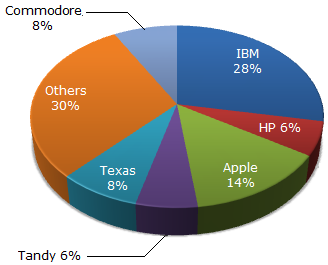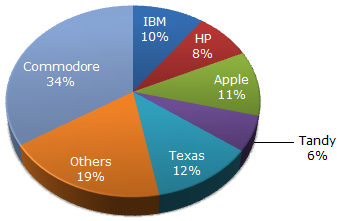# Data Interpretation - Pie Charts - Discussion

The pie chart shows the distribution of New York market share by value of different computer companies in 2005.The pie chart shows the distribution of New York market share by volume of different computer companies in 2005.

Number of units sold in 2005 in New York = 1,500

Value of units sold in 2005 in New York = US \$1,650,000.2.

Over the period 2005-2006, if sales (value-wise) of IBM PC's increased by 50% and of Apple by 15% assuming that PC sales of all other computer companies remained the same, by what percentage (approximately) would the PC sales in New York (value-wise) increase over the same period ?

 [A]. 16.1 % [B]. 18 % [C]. 14 % [D]. None of these

Explanation:

If we assume the total sales to be 100 in the first year, IBM's sales would go up by 50% (from 28 to 42) contributing an increase of 14 to the total sales value.

Similarly, Apple's increase of 15% would contribute an increase of 2.1 to the total sales value. The net change would be 14 + 2.1 on 100. (i.e., 16.1%)

 Varun said: (Oct 22, 2011) Can any one can explain me this question in detail please?

 Joy Broto Nath said: (Oct 23, 2011) I cannot understand how IBM sales if go up by 50% contribute to increase of 14 to total sales?

 Anoop said: (Oct 25, 2011) Total sales in value in 2005 = US \$1,650,000. Since this is all about percentage the value doesn't matter. So we assume the total sales in value in 2005 = US\$100(instead of 1650000) In 2005 IBM's contribution was 28% = \$28(in cash) IBM got 50% increase in sales ie \$14 (14% of 100) In 2005 Apples's contribution was 14% = \$14(in cash) Apple got 15% increase in sales ie \$ \$2.1 (2.1% of 100) The net increase in sales = 14+2.1 =16.1 %ge increase in sales = 16.1*100/100 = 16.1

 Zahid said: (Sep 24, 2013) @Anoop. From where did you get 14 and 2.1 ?

 Avani said: (Oct 13, 2014) IBM PC increased by 50% that is (28*150)/100 = 42. So here increase is 42-28 = 14. Apple increased by 15 % that is (14*115)/100 = 16.1. So here increase is 16.1-14 = 2.1. Hence total increase 14+2.1 = 16.1.

 Chidre said: (Oct 13, 2015) Here 50% of IBM sales is increased okay but why you are taking 50% on 28 instead of 10%? Because 1st chart is of market share, second chart is of sales.

 Reena said: (Jun 7, 2016) Because they have specified it to be in terms of value.

 Shivani said: (Oct 22, 2016) Please explain the method to solve the question.

 Inayat said: (Dec 7, 2016) The answer should be D here "None of these". The chart shows market share in %, all percentages together is 100%. Somehow total share should not exceed more than 100%. All companies together market share should be 100%. In this case, when sale % increases market share is gone to 116.1% which will not be correct. If you want to balance, other companies market share to be reduced to balance to 100%. So the answer can be D. If I'm wrong please explain me in a little better way how the answer is A?

 Divya Mohana said: (Mar 1, 2017) From where did 28 come?

 Bill said: (Mar 29, 2017) Thanks @Anoop & @Avani.

 Bhaskar said: (Jun 17, 2017) Thanks @Anoop.

 Archana said: (Jul 8, 2017) Thanks @Anoop and @Avani.

 Amisha said: (May 21, 2018) Thanks @Avani.

 Ashwini said: (Jun 18, 2018) Can you please explain how did you get 150 and 115?

 Nafeesa said: (Oct 19, 2018) @All. 50% increase means 150 (50+100) and 15% means 115.

 Purnima said: (Apr 29, 2020) Thanks @Nafeesa.

 Purnima said: (Apr 29, 2020) Thank you @Avani.

 Moon said: (Jun 1, 2020) Thanks @Avani.

 Harry said: (Jun 4, 2020) At last step, why did you do multiplication with 100 and divide 100 with 16.1?

 Barac said: (Jan 22, 2021) 50% of 28 = 28*(50/100) = 14, 15%of 14=14*(15/100) = 2.1. Therefore total increased = 14+2.1 = 16.1.

 Raja said: (Aug 5, 2021) @Avani. Good explanation. Thank you.

 Mariya said: (Aug 25, 2021) Excellent explanation, Thank you @Avani.